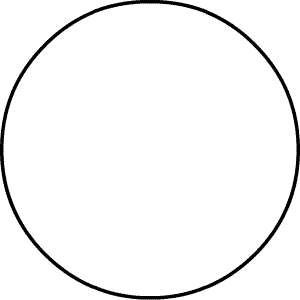Skill 5H
Make a Circle Graph

Follow the directions to make a circle graph.

 Favorite Food Food Number of Students Pizza 5 Hamburgers 2 Tacos 31. What is the sum of the column labeled "Number of Students"? _____

2. Divide the circle graph into equal parts using the total sum that you found from instruction number one above.

3. Color your circle graph. Use a different color for each food.

4. Label each food on the circle graph.

5. Write a title above the circle graph.